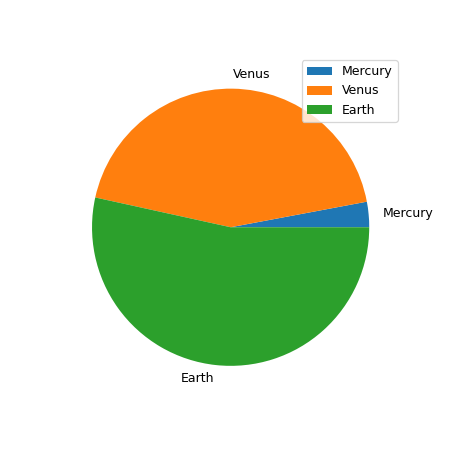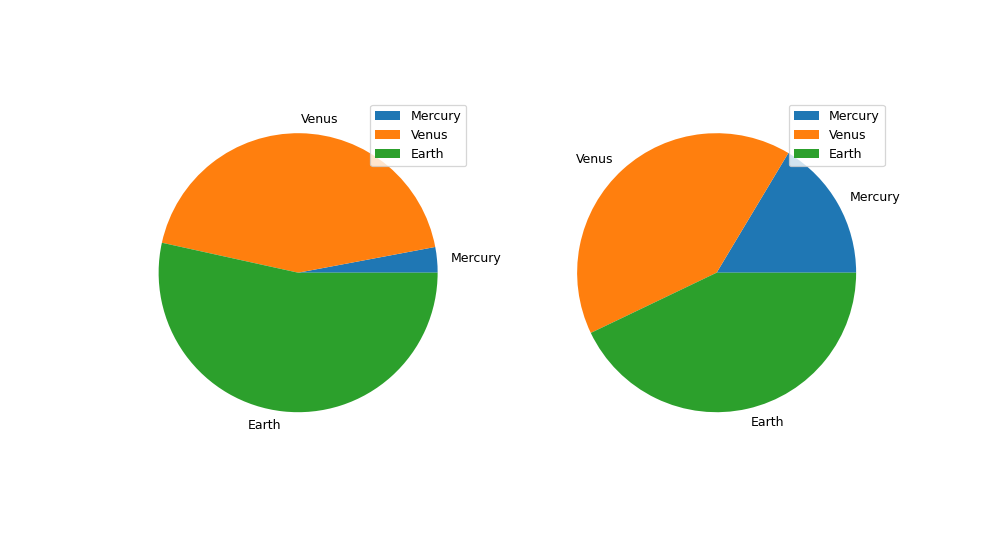# pandas.Series.plot.pie¶

Series.plot.pie(**kwargs)[source]

Generate a pie plot.

A pie plot is a proportional representation of the numerical data in a column. This function wraps matplotlib.pyplot.pie() for the specified column. If no column reference is passed and subplots=True a pie plot is drawn for each numerical column independently.

Parameters
yint or label, optional

Label or position of the column to plot. If not provided, subplots=True argument must be passed.

**kwargs

Keyword arguments to pass on to DataFrame.plot().

Returns
matplotlib.axes.Axes or np.ndarray of them

A NumPy array is returned when subplots is True.

Series.plot.pie

Generate a pie plot for a Series.

DataFrame.plot

Make plots of a DataFrame.

Examples

In the example below we have a DataFrame with the information about planet’s mass and radius. We pass the ‘mass’ column to the pie function to get a pie plot.

>>> df = pd.DataFrame({'mass': [0.330, 4.87 , 5.97],>>> plot = df.plot.pie(subplots=True, figsize=(11, 6))